### Total Pressure and Centre of Pressure

The total pressure is defined as the force exerted by a static fluid on a surface (either plane or curved) when the fluid comes in contact with the surface. This force is always normal to the surface. The centre of pressure is defined as the point of application of the resultant pressure on the surface.

The total pressure and centre of pressure on the immersed surfaces are as follows: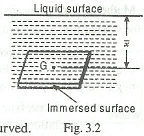1. Horizontally immersed surface. The total pressure on a horizontally immersed surface, as shown in Fig. 3.2, is given by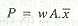where

w = Specific weight of the liquid,

A = Area of the immersed surface, and
x (bar) = Depth of the centre of gravity of the immersed surface from the liquid surface.

The above expression holds good for all surfaces whether flat or curved.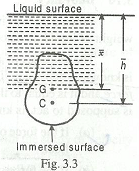2. Vertically immersed surface. The total pressure on a vertically immersed surface, as shown in Fig. 3.3 , is given by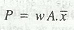and the depth of centre of pressure from the liquid surface,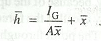where

A = Area of immersed surface,

x (bar) = Depth of centre of gravity of the immersed surface from the liquid surface, and

Ig = Moment of inertia of immersed surface about the horizontal axis through its centre of gravity.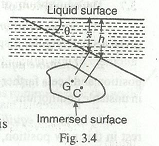3. Inclined immersed surface. The total pressure on an inclined surface, as shown in Fig. 3.4, is given by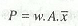and the depth of centre of pressure from the liquid surface,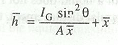where

Theeta = Angle at which the immersed surface is inclined with the liquid surface.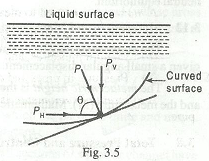4. Curved immersed surface. The total force on the curved surface, as shown in Fig. 3.5, is given by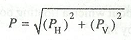and the direction of the resultant force on the curved surface with the horizontal is given by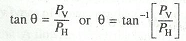where

PH = Horizontal force on the curved surface and is equal to the total pressure on the projected area of the curved surface on the vertical plane, and

Pv = Vertical force on the curved surface and is equal to the weight of the liquid supported by the curved surface upto the liquid surface.

#### 1 comment:

1.Also triangular, rectangular,trapezodialshapes,and circular can wrote sir